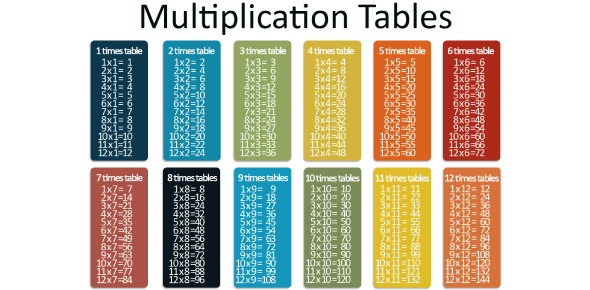Times Table 1-12 Multiplication Quiz!

35 Questions | Total Attempts: 13237SettingsCheck out this superb timetable multiplication quiz that has been created to test your mathematics skills. Are you good at multiplication? Do you know the multiplication table of all the digits? Well, let's find out today. Answer the questions below and see how strong your number game is. The final score will be displayed at the end of this quiz and will reflect how well you performed. Let's start the quiz then.

• 1.
1 x 1 =
• 2.
1 x 2 =
• 3.
1 x 3 =
• 4.
1 x 4 =
• 5.
1 x 5 =
• 6.
1 x 6 =
• 7.
1 x 7 =
• 8.
1 x 8 =
• 9.
1 x 9 =
• 10.
1 x 10 =
• 11.
1 x 11 =
• 12.
1 x 12 =
• 13.
2 x 1 =
• 14.
2 x 2 =
• 15.
2 x 3 =
• 16.
2 x 4 =
• 17.
2 x 5 =
• 18.
2 x 6 =
• 19.
2 x 7 =
• 20.
2 x 8 =
• 21.
2 x 9 =
• 22.
2 x 10 =
• 23.
2 x 11 =
• 24.
2 x 12 =
• 25.
3 x 1 =
Related TopicsBack to top